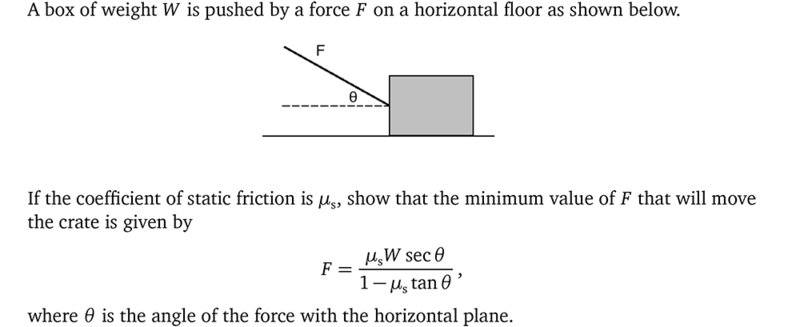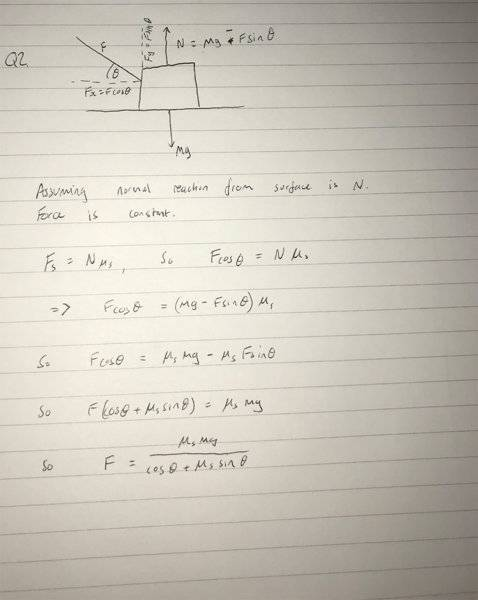# What is the minimum force at this angle that will move the box?

zehkari

## Homework Statement

[/B]2. Homework Equations

Fs = Nμ
W = mg
Fy = Fsin(θ)
Fx = Fcos(θ)

## The Attempt at a Solution

[/B]
Hello all at PhysicsForums,

I have a question on angled force and static friction. From my attempt below, I think I have found the maximum force required and would need to get the minimum. Then using trigonometry I could derive sec(θ) and tan(θ).

Have tried some algebra to get to the quation in the question, but I have been unsuccessful.

If anyone could help me that would be great,
Thanks.
Zehkari.#### Attachments

Last edited by a moderator:

Mentor
Hint: How might that cos(θ) in the denominator become a 1?

zehkari
Something with using the identity
cos2(θ)+sin2(θ) = 1
so
1 - sin2(θ) = cos2(θ)

Mentor
Something with using the identity
cos2(θ)+sin2(θ) = 1
so
1 - sin2(θ) = cos2(θ)
Nope. even simpler.

zehkari
By dividing by itself? -_-

By dividing by itself? -_-
Try that!

zehkari
Try that!

Dividing the euqation by cos(θ), I get:

F/cos(θ) = mg.μ/1+μ.tan(θ)

so,

F = mg.μ.cos(θ)/1+μ.tan(θ)

Mentor
I just noticed that you have a sign error in the denominator of your solution attempt. The μssin(θ) term should have ended up negative. You'll have to go through your work again to find out where the sign error occurred.
Dividing the euqation by cos(θ), I get:

F/cos(θ) = mg.μ/1+μ.tan(θ)
No, check your algebra. I'd suggest manipulating only the right-hand side, leaving the left alone. Divide the numerator and denominator by cos(θ) (hence effectively multiplying the right-hand side by 1).

Dividing the euqation by cos(θ), I get:

F/cos(θ) = mg.μ/1+μ.tan(θ)

so,

F = mg.μ.cos(θ)/1+μ.tan(θ)

Please check your work, before this last step. For example, check the line you wrote for F cosθ = (mg - F sinθ) μs

zehkari
Thank you gneill and Chandra Prayaga, makes sense now. Apologies for the silly error on the sign.

Mentor
Thank you gneill and Chandra Prayaga, makes sense now. Apologies for the silly error on the sign.
I hope that you've reached the desired result? Otherwise, please post your work and we'll be happy to offer more help.

Homework Helper
Gold Member
2021 Award
You'll have to go through your work again to find out where the sign error occurred.
The error is in the diagram that shows N=mg - μkF sinθ. Because the external force is pushing down and across, the reaction force should be greater than mg, not less.

•gneill
zehkari
I hope that you've reached the desired result? Otherwise, please post your work and we'll be happy to offer more help.

I think its good now. As the numerator is divided by cos(θ), you get sec(θ). Then as the denominator is divided by cos(θ), you get 1-μ.tan(θ). Thank you for your help.

The error is in the diagram that shows N=mg - μkF sinθ. Because the external force is pushing down and across, the reaction force should be greater than mg, not less.

Thank you, yes I have it as greater than now.

•gneill